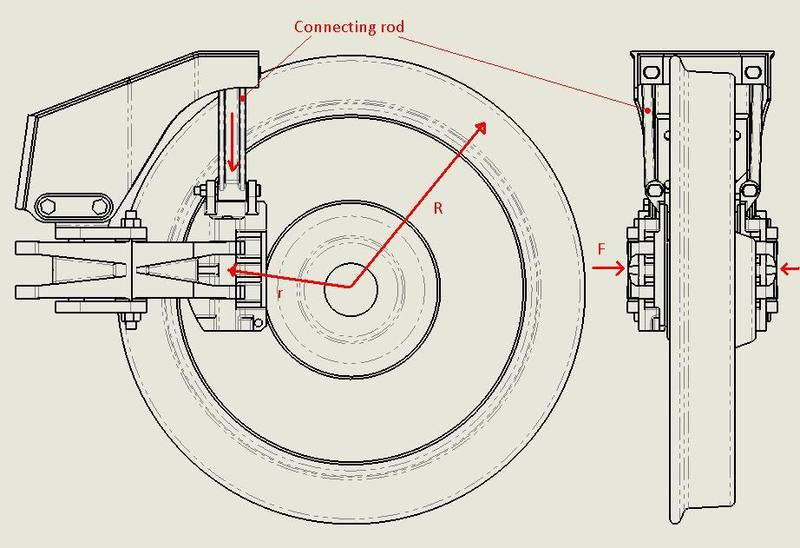# Force on a connecting rod

pibot

## Homework Statement

what is the down force on the connecting rod?

i tinnk ive work most of it but i'm a bit unsure of what to do next?A train of mass 138800kg
have 24 wheels (12 axles)
V = 37.1 m/s
U =0
s= 694 m
F= 12.1KN
R = 0.4 m
r = 0.21 m

## Homework Equations

V2 = U2 + 2 x a x s

Average braking force for the whole train = m x a

## The Attempt at a Solution

a = -0.99m/s

Average braking force for the whole train 138800 x -0.99 = 137KN

braking Force for each wheel 137/24 = 5.7KN

so this is were i need the help please

Were is this braking force atchley acting on the wheel ( at the rim the edge of were?)

when the force F is clamping on the wheel the force from the wheel will transfer to the rod?

I think you have done wrong.Here you need to check torques as it is not point object but a rigid body.

pibot
i did think torques but all i can think is t = f x r

so is this 5.7 x .21 =1.194 KN/M

Whats that in a straght down force on the rod?

Yes it's tangential force.And both torques will get added.

pibot
sorry what do you mean by both will be added?

connecting rods touch disc at two points at r, and R.One is anti-clockwise torque and other is also anti-clockwise.So both get added.That's what it seems if you have both rods touching disc to brake it down.It seems unclear here.Though you haven't stated it here that only one rod touches disc or both do.

pibot
sorry for my unclear pic.

r is the distance from the center of the wheel to the point which the rod acts

R is the effective wheel radius.

the top of the rod it fixed to the braking braket.

the down force on the braket is what is needed?

pibot
Train mass: 96’800KG
Dater source: Angle Trains Web
sitehttp://www.angeltrains.co.uk/en/fleet-portfolio/data-sheets/view/22-br-outer-suburban-emu
Emergency stop test mass: 14’000KG Per Vehicle
Total mass: M = 96’800 +14’000 + 14’000 + 14’000 = 138’800KG
Speed of test: 83MPH = 37.1M/S
Stopping distance: S = 694m
Coefficient of friction between the brake pad and steel disk: µ = 0.46
Number of brake disks on the train: 24
Number of pads per disk: 2
Effective wheel radius: R = 0.42m
Centre of wheel to centre of brake pad: r = 0.21m

Deceleration rate: a = - 1/2 × (V_(t )^2- U_0^2)/S = - 1/2 × (〖37.1〗^2- 0^2)/694
a = -0.99m/s
Time: t = (V_(t )- U_0)/a = (37.1-0)/(-0.99)
t = 37.5 Seconds
Average braking force for the Whole train: F_Average = Mass * Acceleration
: = 138’800 * -0.99
= -137’412N (-137KN)
Average braking force per wheel: = F_Wheel = F_Average/24
= (-137412)/24
= -5725.5N (5.7KN)
Maximum press exerted on the disk by the brake pads: μ×F_Pad = R/r × F_Wheel/2
= 0.42/0.21 × 5.7/2
= 12.4 KN